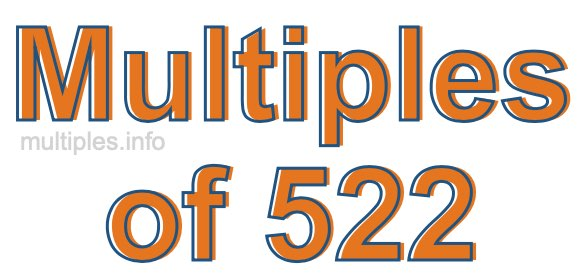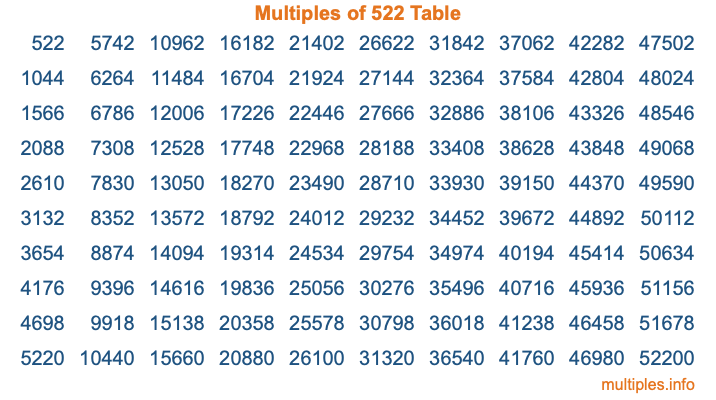Multiples of 522Welcome to the Multiples of 522 page. Here we will first teach you everything you will ever need to know about the multiples of 522, and then give you a study guide summary of everything we taught you to make sure you remember it all. Use this page to look up facts and learn information about the multiples of 522. This page will make you a multiples of five hundred twenty-two expert!

Definition of Multiples of 522
Multiples of 522 are all the numbers that when divided by 522 equal an integer. Each of the multiples of 522 are called a multiple. A multiple of 522 is created by multiplying 522 by an integer.

Therefore, to create a list of multiples of 522, you start with 1 multiplied by 522, then 2 multiplied by 522, then 3 multiplied by 522, and so on for as long as you want. Thus, the list of the first five multiples of 522 is 522, 1044, 1566, 2088, and 2610. To see a larger list of multiples of 522, see the printable image of Multiples of 522 further down on this page. We also have a category where you can choose any nth multiple of 522.

Multiples of 522 Checker
The Multiples of 522 Checker below checks to see if any number of your choice is a multiple of 522. In other words, it checks to see if there is any number (integer) that when multiplied by 522 will equal your number. To do that, we divide your number by 522. If the the quotient is an integer, then your number is a multiple of 522.

Is  a multiple of 522?

Least Common Multiple of 522 and ...
A Least Common Multiple (LCM) is the lowest multiple that two or more numbers have in common. This is also called the smallest common multiple or lowest common multiple and is useful to know when you are adding our subtracting fractions. Enter one or more numbers below (522 is already entered) to find the LCM.

Check out our LCM Calculator if you need more details about the Least Common Multiple or if you need the LCM for different numbers for adding and subtraction fractions.

nth Multiple of 522
As we stated above, 522 is the first multiple of 522, 1044 is the second multiple of 522, 1566 is the third multiple of 522, and so on. Enter a number below to find the nth multiple of 522.

th multiple of 522

Multiples of 522 vs Factors of 522
522 is a multiple of 522 and a factor of 522, but that is where the similarities end. All postive multiples of 522 are 522 or greater than 522. All positive factors of 522 are 522 or less than 522.

Below is the beginning list of multiples of 522 and the factors of 522 so you can compare:

Multiples of 522: 522, 1044, 1566, 2088, 2610, etc.

Factors of 522: 1, 2, 3, 6, 9, 18, 29, 58, 87, 174, 261, 522

As you can see, the multiples of 522 are all the numbers that you can divide by 522 to get a whole number. The factors of 522, on the other hand, are all the whole numbers that you can multiply by another whole number to get 522.

It's also interesting to note that if a number (x) is a factor of 522, then 522 will also be a multiple of that number (x).

Multiples of 522 vs Divisors of 522
The divisors of 522 are all the integers that 522 can be divided by evenly. Below is a list of the divisors of 522.

Divisors of 522: 1, 2, 3, 6, 9, 18, 29, 58, 87, 174, 261, 522

The interesting thing to note here is that if you take any multiple of 522 and divide it by a divisor of 522, you will see that the quotient is an integer.

Multiples of 522 Table
Below is an image of the first 100 multiples of 522 in a table. The table is in chronological order, column by column. The first column has the first ten multiples of 522, the second column has the next ten multiples of 522, and so on.The Multiples of 522 Table is also referred to as the 522 Times Table or Times Table of 522. You are welcome to print out our table for your studies.

Negative Multiples of 522
Although not often discussed or needed in math, it is worth mentioning that you can make a list of negative multiples of 522 by multiplying 522 by -1, then by -2, then by -3, and so on, to get the following list of negative multiples of 522:

-522, -1044, -1566, -2088, -2610, etc.

Multiples of 522 Summary
Below is a summary of important Multiples of 522 facts that we have discussed on this page. To retain the knowledge on this page, we recommend that you read through the summary and explain to yourself or a study partner why they hold true.

There are an infinite number of multiples of 522.

A multiple of 522 divided by 522 will equal a whole number.

522 divided by a factor of 522 equals a divisor of 522.

The nth multiple of 522 is n times 522.

The largest factor of 522 is equal to the first positive multiple of 522.

522 is a multiple of every factor of 522.

522 is a multiple of 522.

A multiple of 522 divided by a divisor of 522 equals an integer.

522 divided by a divisor of 522 equals a factor of 522.

Any integer times 522 will equal a multiple of 522.

Multiples of a Number
Here you can get the multiples of another number, all with the same attention to detail as we did for multiples of 522 on this page.

Multiples of
Multiples of 523
Did you find our page about multiples of five hundred twenty-two educational? Do you want more knowledge? Check out the multiples of the next number on our list!